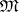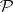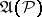# Turing machine

(diff) ← Older revision | Latest revision (diff) | Newer revision → (diff)

The name attached to abstract computers (cf. Computer, abstract) of a specific type. The concept of a machine of such a kind originated in the middle of the 1930's from A.M. Turing

as the result of an analysis carried out by him of the actions of a human being carrying out some or other calculations in accordance with a plan worked out in advance, that is, carrying out successive transformations of complexes of symbols. This analysis, in turn, was carried out by him with the aim of solving the then urgent problem of finding a precise mathematical equivalent for the general intuitive idea of an algorithm. In the course of development of the theory of algorithms (cf. Algorithms, theory of), there emerged a number of modifications of the original definition of Turing. The version given here goes back to E. Post ; in this form the definition of a Turing machine has achieved widespread popularity (the Turing machine has been described in detail, for example, in  and ).

A Turing machine is conveniently represented as an automatically-functioning system capable of being in a finite number of internal states and endowed with an infinite external memory, called a tape. Two of the states are distinguished, the initial state and the final state. The tape is divided into cells and is unbounded to the left and to the right. Any letter of some finite alphabetcan be printed on each cell of the tape (for the sake of uniformity, it is convenient to regard an empty cell as being printed with a "blank" ). At each moment of discrete time the Turing machine is in one of its states, and by scanning one of the cells of its tape it perceives the symbol written there (a letter of the alphabetor the blank).

If the Turing machine is in a non-final state at some moment of time, it completes a step, which is completely determined by its current state and the symbol that is perceived on the tape at this moment. A step consists of the following: 1) print a new symbol in the scanned cell, which may be the same as the old symbol or a blank; 2) go to a new state, which may be the same as the old one or the final state; and 3) move the tape to the left or to the right by one cell, or keep it in the same place. The list of all possible steps of the Turing machine in dependence on the current combination of "non-final state + symbol perceived" can be represented, for example, by a special table with two inputs, called the program, or scheme, of the given Turing machine. The codes of the corresponding steps of the machine, called its commands, are placed in the cells of this table. The program of the Turing machine is an object with a given structure, and one can stipulate that the Turing machine be identified with its program. If one wants to emphasize the connection of such a Turing machine with the alphabet, then one usually says that this machine is a Turing machine in the alphabet.

The complete description of the current state of a Turing machine is given by its configuration, consisting of the following information at the given moment: 1) the actual symbols filling the cells of the tape; 2) the cell currently being scanned by the machine; and 3) the internal state of the machine. A configuration corresponding to the final state of the Turing machine is also called final.

If some non-final configuration of the Turing machine is fixed as the initial configuration, then the functioning of this machine will consist of a (step by step) sequential transformation starting with the initial configuration in accordance with the machine's program until the time of attaining a final configuration. After this, the functioning of the Turing machine is considered ended and the final configuration attained is regarded as the result of the functioning of the machine. Of course, the functioning of the Turing machine does not, in general, terminate for every initial configuration.

The notion of a Turing machine can be used for making precise the general idea of an algorithm in a given alphabet, as follows. By a Turing algorithm in an alphabetis meant any algorithmof the following kind. One takes a fixed Turing machinein the alphabet. Letbe the word intaken as the initial data for the algorithm. The following initial configuration of the machineis constructed: 1) the wordis written on the tape without gaps, the remaining cells being left empty (i.e. blank); 2) the machineis set up to scan the cell with the first letter of the word; and 3)is put into the initial state (ifis empty, then the tape is chosen to be empty, and the scanned cell is any cell). Suppose that, starting from this initial configuration, completes its functioning. Consider the cell of the tape being scanned byin the final configuration. If the symbol printed on it is blank, thenis taken to be the empty word. Otherwise,is taken to be the word printed on the maximum segment of the tape including the scanned cell and not containing any blanks.

There are strong grounds for supposing that the precise description of the general idea of an algorithm in an alphabet carried out by means of the notion of a Turing machine is adequate. Namely, it is held that for every algorithmin some alphabet it is possible to construct a Turing algorithm giving the same results under the same initial data as the algorithm. This convention is known in the theory of algorithms as the Turing thesis. The acceptance of the Turing thesis is equivalent to the acceptance of the Church thesis (for partial recursive functions) or the normalization principle (for normal algorithms, cf. Normal algorithm). However, in contrast to the latter two, the Turing thesis is immediately highly convincing. In fact, by carrying out computations according to a selected plan, the mathematician acts in a way similar to a Turing machine: in considering some position in his writings and being in a certain "state of mind" , he makes the necessary alterations in his writing, is inspired by a new "state of mind" , and goes on to contemplate further writing. The fact that he completes more complicated steps than a Turing machine seems not principally significant.

In terms of the structure of their description and the type of functioning, Turing machines are automata of a very general kind, so that Turing's conception has to a considerable extent stimulated the origin of the abstract theory of automata and largely predetermined their particular properties (cf. Automaton; Automata, theory of).

There are many modifications of Turing machines. The most widespread are multi-tape Turing machines, with one or several heads for each of its tapes. The motion of the heads and the printing of the letters on the tape are carried out simultaneously according to the program of the control system. Multi-tape Turing machines are conveniently used in the formalization of the notion of a relative algorithm. Thus, a functionis (algorithmically) computable relative to a functionif there exists a multi-tape Turing machine that computesunder the condition that in any initial configuration all the values ofare printed in fixed order on one of the tapes. In this form one can, in terms of relative computations, introduce the important notion of Turing reducibility in the theory of algorithms, as well as other forms of algorithmic reducibility. It is natural to formalize the concept of a probabilistic algorithm by means of multi-tape Turing machines. A common approach consists of the following: A random sequence is printed on one of the tapes of the multi-tape Turing machine; the Turing machine then deals with exactly one symbol of this sequence at each instant. In a second approach, the program of the control system of the Turing machine will allow the existence of several commands with the same left-hand sides, the choice of one or other of the commands then being carried out with prescribed probabilities. The notion of a non-deterministic Turing machine is based on a similar idea. Here again, the program of the control system can have several commands with the same left-hand sides. In both cases, instead of a single computation for a given input, one considers the class of all possible computations compatible with the program. For probabilistic Turing machines the probability of such computations is considered; for non-deterministic Turing machines one considers the possibility of the computation itself.

How to Cite This Entry:
Turing machine. Encyclopedia of Mathematics. URL: http://encyclopediaofmath.org/index.php?title=Turing_machine&oldid=17566
This article was adapted from an original article by N.M. NagornyiS.S. Marchenkov (originator), which appeared in Encyclopedia of Mathematics - ISBN 1402006098. See original article